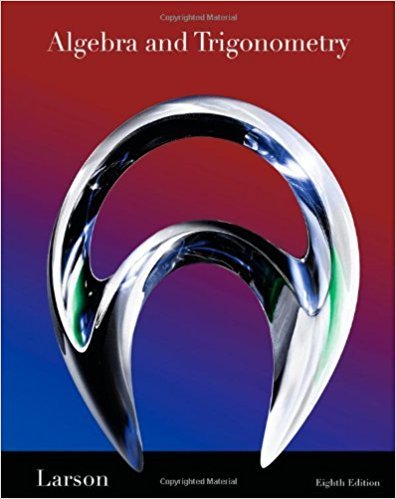×
×

# Solutions for Chapter P.3: Polynomials and Special Products## Full solutions for Algebra and Trigonometry | 8th Edition

ISBN: 9781439048474Solutions for Chapter P.3: Polynomials and Special Products

Solutions for Chapter P.3
4 5 0 405 Reviews
11
5
##### ISBN: 9781439048474

This expansive textbook survival guide covers the following chapters and their solutions. This textbook survival guide was created for the textbook: Algebra and Trigonometry, edition: 8. Algebra and Trigonometry was written by and is associated to the ISBN: 9781439048474. Chapter P.3: Polynomials and Special Products includes 127 full step-by-step solutions. Since 127 problems in chapter P.3: Polynomials and Special Products have been answered, more than 39659 students have viewed full step-by-step solutions from this chapter.

Key Math Terms and definitions covered in this textbook
• Big formula for n by n determinants.

Det(A) is a sum of n! terms. For each term: Multiply one entry from each row and column of A: rows in order 1, ... , nand column order given by a permutation P. Each of the n! P 's has a + or - sign.

• Eigenvalue A and eigenvector x.

Ax = AX with x#-O so det(A - AI) = o.

• Fibonacci numbers

0,1,1,2,3,5, ... satisfy Fn = Fn-l + Fn- 2 = (A7 -A~)I()q -A2). Growth rate Al = (1 + .J5) 12 is the largest eigenvalue of the Fibonacci matrix [ } A].

• Hilbert matrix hilb(n).

Entries HU = 1/(i + j -1) = Jd X i- 1 xj-1dx. Positive definite but extremely small Amin and large condition number: H is ill-conditioned.

• Hypercube matrix pl.

Row n + 1 counts corners, edges, faces, ... of a cube in Rn.

• Identity matrix I (or In).

Diagonal entries = 1, off-diagonal entries = 0.

• Kirchhoff's Laws.

Current Law: net current (in minus out) is zero at each node. Voltage Law: Potential differences (voltage drops) add to zero around any closed loop.

• lA-II = l/lAI and IATI = IAI.

The big formula for det(A) has a sum of n! terms, the cofactor formula uses determinants of size n - 1, volume of box = I det( A) I.

• Least squares solution X.

The vector x that minimizes the error lie 112 solves AT Ax = ATb. Then e = b - Ax is orthogonal to all columns of A.

• Normal equation AT Ax = ATb.

Gives the least squares solution to Ax = b if A has full rank n (independent columns). The equation says that (columns of A)·(b - Ax) = o.

• Pivot.

The diagonal entry (first nonzero) at the time when a row is used in elimination.

• Positive definite matrix A.

Symmetric matrix with positive eigenvalues and positive pivots. Definition: x T Ax > 0 unless x = O. Then A = LDLT with diag(D» O.

• Projection matrix P onto subspace S.

Projection p = P b is the closest point to b in S, error e = b - Pb is perpendicularto S. p 2 = P = pT, eigenvalues are 1 or 0, eigenvectors are in S or S...L. If columns of A = basis for S then P = A (AT A) -1 AT.

• Rank r (A)

= number of pivots = dimension of column space = dimension of row space.

• Row picture of Ax = b.

Each equation gives a plane in Rn; the planes intersect at x.

• Schwarz inequality

Iv·wl < IIvll IIwll.Then IvTAwl2 < (vT Av)(wT Aw) for pos def A.

• Solvable system Ax = b.

The right side b is in the column space of A.

• Spectral Theorem A = QAQT.

Real symmetric A has real A'S and orthonormal q's.

• Vector space V.

Set of vectors such that all combinations cv + d w remain within V. Eight required rules are given in Section 3.1 for scalars c, d and vectors v, w.

• Volume of box.

The rows (or the columns) of A generate a box with volume I det(A) I.

×